# SHSAT Math: Properties of Functions Chapter Exam

Exam Instructions:

Choose your answers to the questions and click 'Next' to see the next set of questions. You can skip questions if you would like and come back to them later with the yellow "Go To First Skipped Question" button. When you have completed the practice exam, a green submit button will appear. Click it to see your results. Good luck!

### Page 1

#### Question 1 1. Using the graph, find f(f(-2)).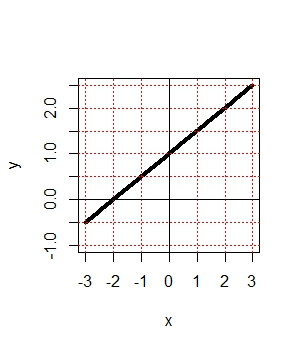### Page 3

#### Question 13 13. Evaluate f(f(x)). [Yes, it says f(f(x)]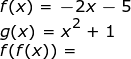### Page 4

#### Question 16 16. Which kind of transformation would change the purple function below into the yellow one?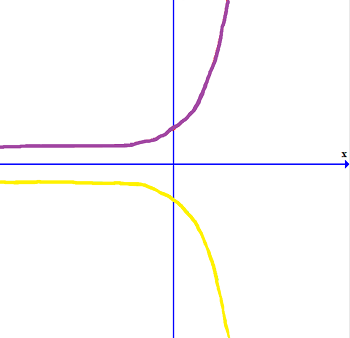#### Question 17 17. Use the graph to find f(f(2)).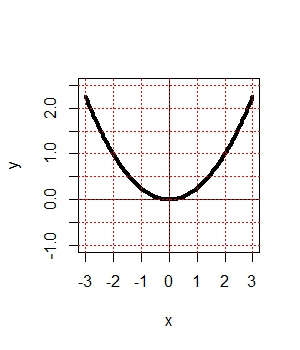#### Question 18 18. Evaluate.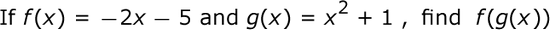#### Question 20 20. What is the range of the function represented by the graph below?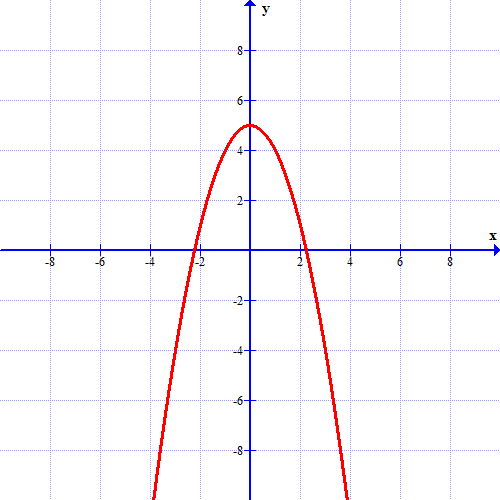### Page 5

#### Question 25 25. The revenue of a company is represented by R(x) and their costs are represented by C(x). What is the profit function R(x) - C(x)?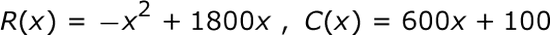### Page 6

#### Question 29 29. If the graph of f(x) is shown below, which choice represents the graph of f(x + 1) + 2?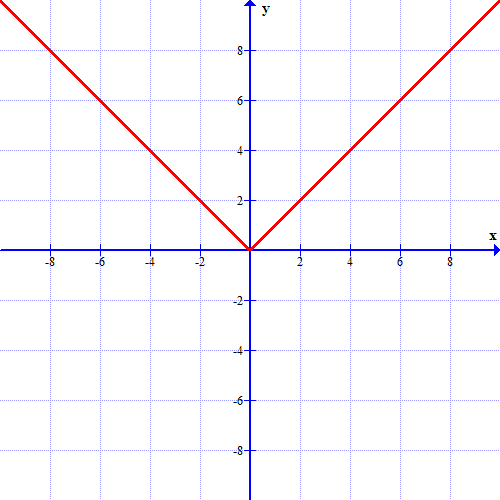#### SHSAT Math: Properties of Functions Chapter Exam Instructions

Choose your answers to the questions and click 'Next' to see the next set of questions. You can skip questions if you would like and come back to them later with the yellow "Go To First Skipped Question" button. When you have completed the practice exam, a green submit button will appear. Click it to see your results. Good luck!

Support